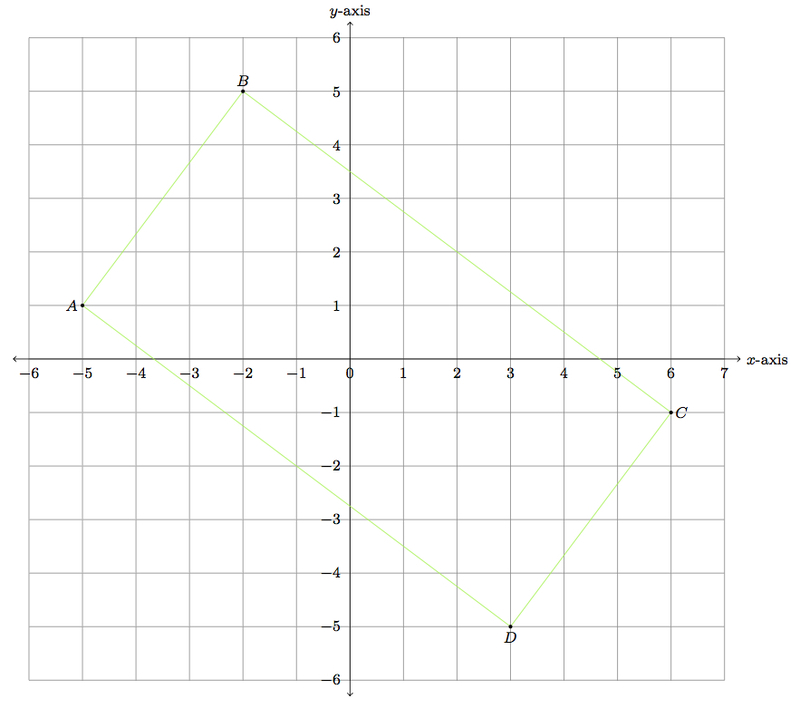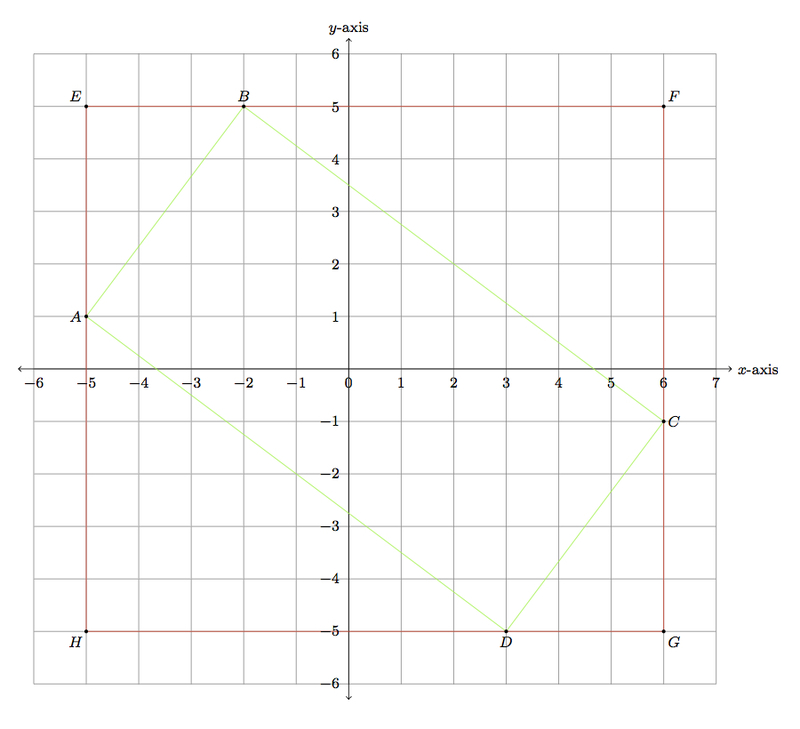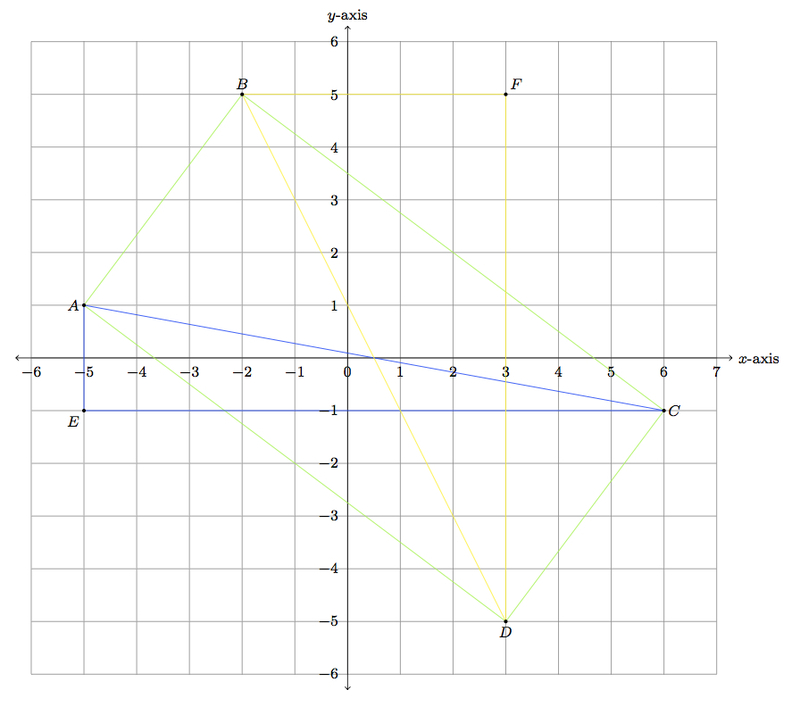# A rectangle in the coordinate plane

Alignments to Content Standards: 8.G.B

Below is a quadrilateral in the coordinate plane:1. Use the Pythagorean theorem to find the lengths of sides $\overline{AB}$, $\overline{BC}$, $\overline{CD}$, and $\overline{DA}$. Can you conclude that quadrilateral $ABCD$ is a rectangle?
2. Use the Pythagorean theorem to find the lengths of the two diagonals $\overline{AC}$ and $\overline{BD}$
3. Use parts (a) and (b) to explain why quadrilateral $ABCD$ is a rectangle.

## IM Commentary

This task provides an opportunity to apply the Pythagorean theorem to multiple triangles in order to determine the length of the hypotenuse; the converse of the Pythagorean theorem is also required in order to conclude that certain angles are right angles. In parts (a) and (b), the Pythagorean theorem can be used to find the different segment lengths because the lines of the coordinate grid make right angles where they meet. These coordinates are essential in order to measure the distance between points joined by horizontal or vertical lines. Part (c) of this problem uses the converse of the Pythagorean theorem: if the sum of the squares of two side lengths of a triangle is equal to the square of the third side length, then the triangle must be a right triangle.

The task can be preceded or followed up by a prompt for students to look for rectangles whose vertices lie at the intersection of the grid lines (other than the ones whose sides are contained in the grid lines). There are many examples. It is difficult, however, to find examples where the side lengths of the rectangle are all whole numbers as is the case for $ABCD$ in this problem.

This task also relates to the standard 8.G.2. Rectangle $ABCD$ is congruent to any $5$ by $10$ rectangle with vertices at the intersection of vertical and horizontal grid lines. To show this congruence, however, requires either a rotation or a reflection. In the former case, finding the number of degrees of the rotation is difficult (as is showing that the rotation has the desired impact on the vertices) while in the latter case finding the equation of the line of reflection is a challenge. This provides motivation for eventually developing congruence criteria such as SSS, SAS, and ASA for triangles.

The diagonals of $ABCD$ each have the same length, $\sqrt{125}$, but the two right triangles which have these diagonals as hypotenuse are not congruent (see the blue and yellow triangles in part (b) of the solution). The Pythagorean theorem, applied to these two triangles gives

\begin{align} 125 &= 10^2 + 5^2\\ 125 &= 11^2 + 2^2. \end{align}

So rectangles which fit in a skew way into the coordinate grid are related to writing whole numbers as sums of squares in two different ways.

This task is primarily intended for instruction purposes. An open-ended version, with slightly different coordinates, is presented in the task ''Is this a rectangle?''

There is an open ended version of this task as well; see 8.G, G-GPE, G-SRT, G-CO Is this a rectangle?

## Solution

1. To help compute the side lengths for quadrilateral $ABCD$ we add rectangle $EFGH$ shown in red in the picture below:The grid lines meet in right angles so $\triangle AEB$, $\triangle BFC$, $\triangle CGD$, and $\triangle DHA$ are all right triangles. Using the Pythagorean theorem, we can find the side lengths for each hypotenuse in these right triangles:

\begin{align} |AB|^2 &= |AE|^2 + |EB|^2 \\ |BC|^2 &= |BF|^2 + |FC|^2 \\ |CD|^2 &= |CG|^2 + |GD|^2 \\ |DA|^2 &= |DH|^2 + |HA|^2 \end{align}

All of the side lengths on the right are whole numbers since these segments are vertical or horizontal with end points having integer coordinates. For example, $A = (-5,1)$ and $E = (-5,5)$ so $|AE| = 4$. Similarly $B = (-2,5)$ and so $|EB| = 3$. Similarly, we find $|BF| = 8, |FC| = 6, |CG| = 4, |GD| = 3, |DH| = 8$, and $|HA| = 6$. Plugging in these lengths we find

\begin{align} |AB| &= 5 \\ |BC| &= 10 \\ |CD| &= 5 \\ |DA| &= 10 \end{align}

Although opposite sides of a rectangle are congruent, this is true of any parallelogram, and not all parallelograms are rectangles. In order to conclude that $ABCD$ is a rectangle, we need to show the four angles are right angles.

2. Below is a picture with two points, labelled $E$ and $F$, which will help to find the lengths of the diagonals of $ABCD$.As in part (a), we can find the lengths of $\overline{AC}$ and $\overline{BD}$ applying the Pythagorean theorem to the right triangles $AEC$ and $DFB$.

\begin{align} |AC|^2 &= |AE|^2 + |EC|^2 \\ |DB|^2 &= |DF|^2 + |FB|^2 \end{align}

As in part (a), the lengths on the right can all be calculated by using the coordinates. So $|AC| = 2$, $|EC| = 11$, $|DF| = 10$, and $|FB| = 5$. Plugging these values into the above equations we find $$|AC| = |DB| = \sqrt{125}.$$

3. To show that $ABCD$ is a rectangle, we need to show that the four angles are right angles. To do this, we use the converse of the Pythagorean theorem. We have

\begin{align} |AC|^2 &= 125 \\ &= 100 + 25\\ &= |AD|^2 + |DC|^2 \\ &= |BC|^2 + |AB|^2 \end{align}

By the converse of the Pythagorean theorem $\triangle ABC$ and $\triangle ADC$ are right triangles and $\angle B$ and $\angle D$ of quadrilateral $ABCD$ are right angles.

Similarly we have

\begin{align} |BD|^2 &= 125 \\ &= 100 + 25\\ &= |AD|^2 + |AB|^2 \\ &= |BC|^2 + |CD|^2 \end{align}

By the converse of the Pythagorean theorem $\triangle BAD$ and $\triangle BCD$ are right triangles and $\angle A$ and $\angle C$ of quadrilateral $ABCD$ are right angles. Thus we have shown that all four angles of $ABCD$ are right angles and so $ABCD$ is a rectangle.